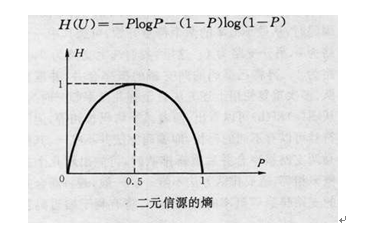# 随机变量的熵的定义与计算公式

### 熵的定义

$f(P)=log(\frac {1}{P}) = -log(P)$

P表示概率

### 离散型随机变量的熵

$H(U) = -\sum_{i=1}^n P_i *log(P_i)$

### 连续型随机变量的熵

$H(U) = -Plog(P) - (1-P)log(1-P)$

U表示随机变量;
P表示随机变量取值为U的概率, 也可以表示为P(U);

### 熵的函数曲线1. 非负性：即收到一个信源符号所获得的信息量应为正值，H(U)≥0;
2. 对称性：即对称于P=0.5;
3. 确定性：H(1,0)=0，即P=0或P=1已是确定状态，所得信息量为零;
4. 极值性：因H(U)是P的上凸函数，且一阶导数在P=0.5时等于0，所以当P=0.5时，H(U)最大。©️2019 CSDN 皮肤主题: 大白 设计师: CSDN官方博客